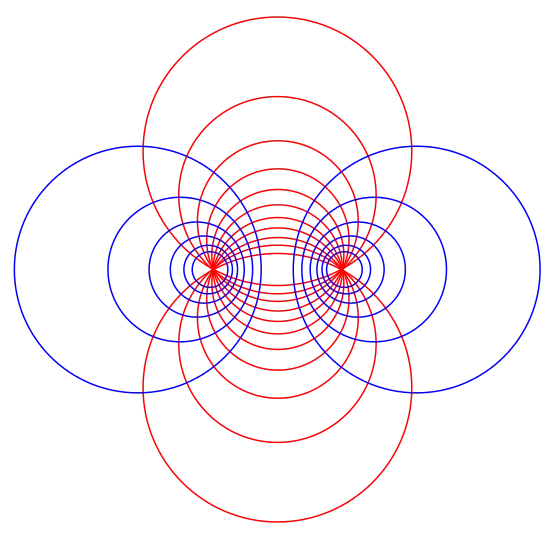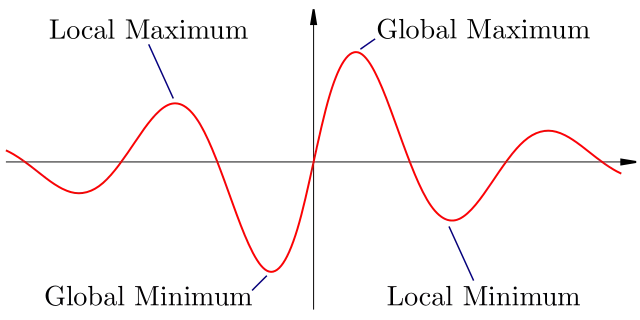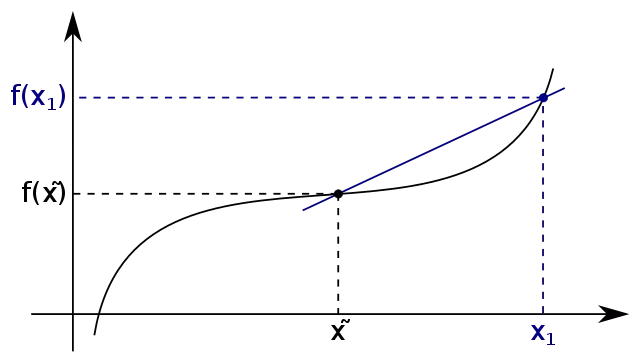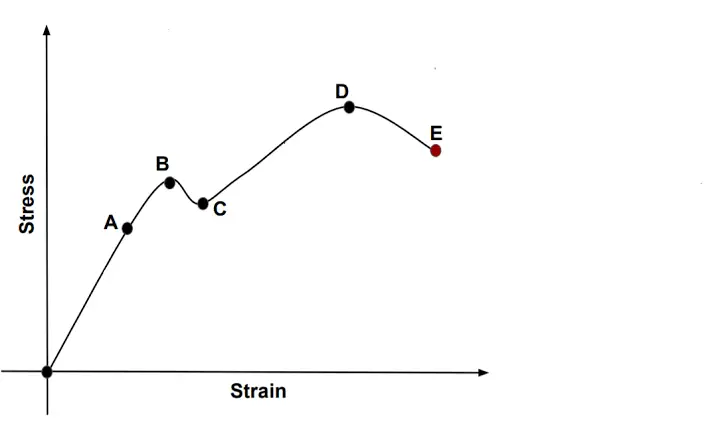# Category IIT – JEE

## Differential Equations Class 12 notes | IIT-JEE study materialSo, in calculus we are starting the topic of differential equations, we will be seeing what does a differential equation exactly means, what are partial differential equations, the significance of differential equations in various disciplines is very vast, we will…

## Applications of Derivatives class 12 revision notes | IIT-JEE notes, study materialWe have analyzed the concept of derivative/Differentiation of a function, this concept is widely used in various fields like math, physics, engineering sciences, etc, derivatives are used to analyze any function by calculating its first and second derivative, Hence we…

## Methods of Differentiation | Concept Booster IIT-JEE Class 12 notesMethods of Differentiation Here we will learn methods of Differentiation, We had already studied the two meanings of derivative(differentiation) of a function which is the geometrical and physical meanings. Since we know what does the differentiation of a function means…

## Differentiability Class 12 | IIT JEE Notes, Concept BoosterDifferentiability The concept of the differentiability (derivability) of a function tells us about whether a given function will be differentiable or not. If the function is differentiable then we will use Methods of differentiation to differentiate a function. Actually, this…

## Functions | Concept Booster, IIT JEE, Class 12 notesKnow Functions, Types of Functions, What is Composite Function ? Periodic Functions ? Properties of functions. You should learn set theory and relations before learning about functions. Functions A function defined from set A to set B is a special…

## Hooke’s Law, Modulus of Elasticity, Stress-strain curve Class 11Here we will learn the modulus of Elasticity, stress Strain Curve, and Hooke’s law of Elasticity. We know that when any force causes deformation to a body the body tries to regain its original configuration. The body which fully regains…